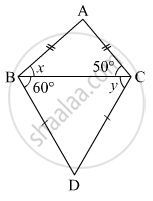Advertisement Remove all ads

# Find the Values of X and Y Using the Information Shown in Figure . - Geometry

Sum

Find the values of x and y using the information shown in figure .Find the measure of ∠ ABD and m∠ ACD.

Advertisement Remove all ads

#### Solution

In △ABC, AB = AC
∴ ∠ABC = ∠ACB            (Angles opposite to equal sides are equal)
⇒ x = 50
In △BDC, DB = DC
∴ ∠DBC= ∠DCB            (Angles opposite to equal sides are equal)
⇒ y = 60
Now, ∠ABD = ∠ABC + ∠DBC
= 50 + 60
= 110
Also, ∠ACD = ∠ACB + ∠DCB
= 50 + 60
= 110
Hence, the values of x and y are 50∘ and 60respectively.

Is there an error in this question or solution?
Advertisement Remove all ads

#### APPEARS IN

Balbharati Mathematics 2 Geometry 9th Standard Maharashtra State Board
Chapter 3 Triangles
Practice Set 3.3 | Q 1 | Page 38
Advertisement Remove all ads
Advertisement Remove all ads
Share
Notifications

View all notifications

Forgot password?